Next: Branch Current Method Up: Kirchoff's Laws Previous: Voltage Divider

## Current Divider

Consider the current divider shown in figure 1.3b. The source current is divided between the two resistors and is given by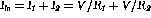. The voltage at the output is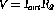. The output current from the current divider is thus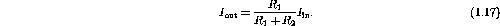Example: Determine an expression for the current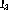through the resistor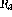in the circuit shown in figure 1.5.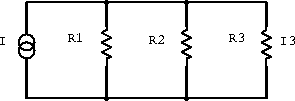Figure 1.5:  Example current divider.

The current I is divided amongst the three resistors and hence we use our expression for resistors in parallel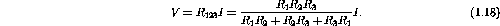where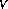is the common voltage across the three parallel resistors. The current throughis thus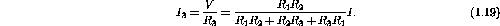Now lets consider some general approaches to solving for unknowns in circuits.

Doug Gingrich
Tue Jul 13 16:55:15 EDT 1999